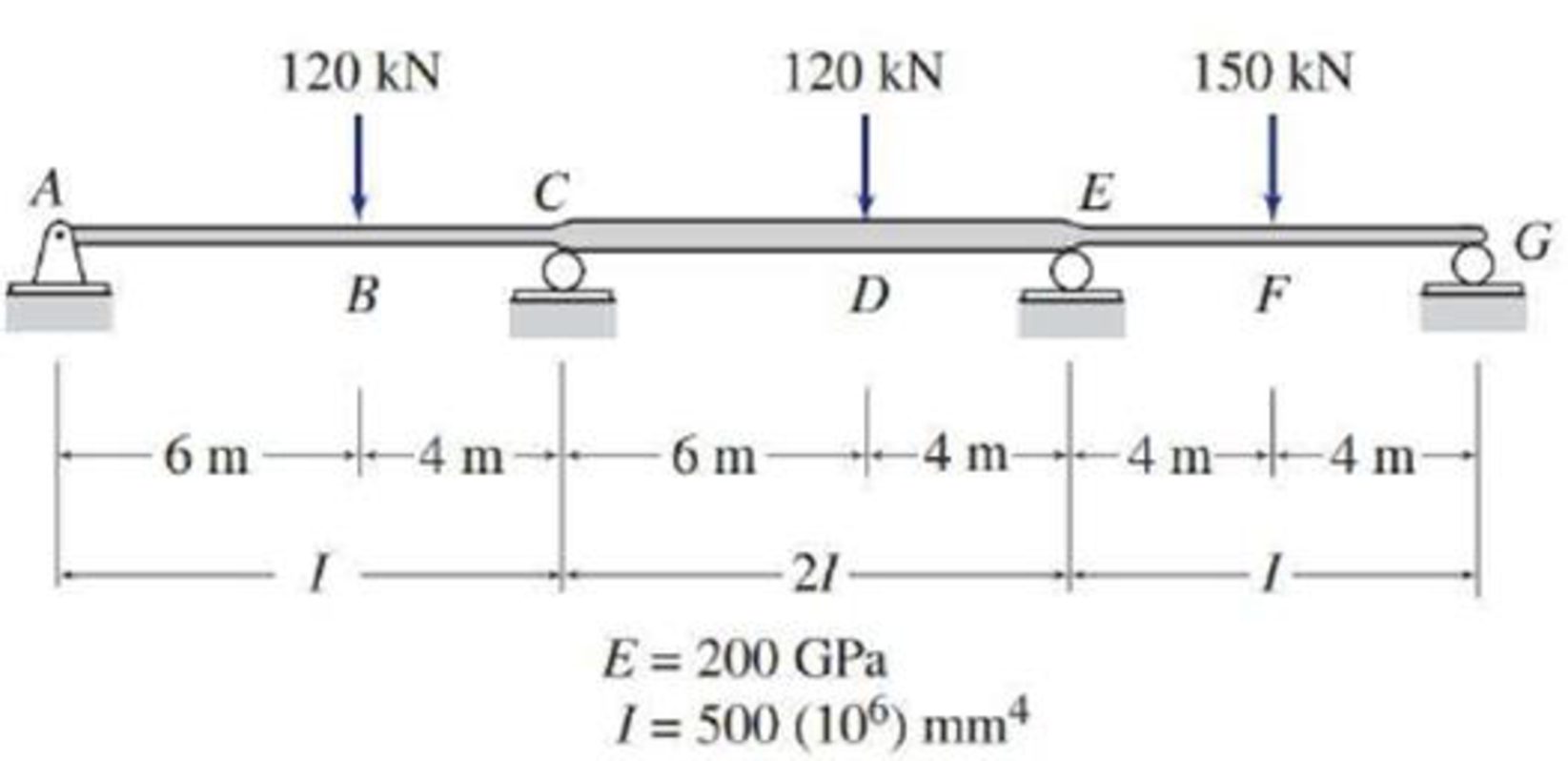# 13.37 through 13.45 Determine the reactions and draw the shear bending moment diagrams for the structures shown in Figs. P13.37–P13.45 using the method of consistent deformations. FIG.P13.39, P13.54

#### Solutions

Chapter
Section
Chapter 13, Problem 39P
Textbook Problem
32 views

## 13.37 through 13.45 Determine the reactions and draw the shear bending moment diagrams for the structures shown in Figs. P13.37–P13.45 using the method of consistent deformations.FIG.P13.39, P13.54

To determine

Calculate the support reactions for the given beam using method of consistent deformation.

Sketch the shear and bending moment diagrams for the given beam.

### Explanation of Solution

Given information:

The structure is given in the Figure.

Apply the sign conventions for calculating reactions, forces and moments using the three equations of equilibrium as shown below.

• For summation of forces along x-direction is equal to zero (Fx=0), consider the forces acting towards right side as positive (+) and the forces acting towards left side as negative ().
• For summation of forces along y-direction is equal to zero (Fy=0), consider the upward force as positive (+) and the downward force as negative ().
• For summation of moment about a point is equal to zero (Matapoint=0), consider the clockwise moment as negative and the counter clockwise moment as positive.

Calculation:

Find the degree of indeterminacy of the structure:

Degree of determinacy of the beam is equal to the number of unknown reactions minus the number of equilibrium equations.

The beam is supported by 5 support reactions and the number of equilibrium equations is 3.

Therefore, the degree of indeterminacy of the beam is i=2.

Select the bending moments at the supports E and C as redundant.

Let θCL and θCR are the slopes at the ends C of the left and right spans of the primary beam due to external loading.

Let θEL and θER are the slopes at the ends E of the left and right spans of the primary beam due to external loading.

Let fCCL and fCCR are the flexibility coefficient representing the slopes at C of the left and right spans due to unit value of redundant MC.

Let fEEL and fEER are the flexibility coefficient representing the slopes at E of the left and right spans due to unit value of redundant ME.

Let fCE be the flexibility coefficient representing the slope at point C of the primary beam due to unit value at point E.

Use beam deflection formulas:

Find the value of θCL using the relation:

θCL=Pa(L2a2)6EIL

Substitute 120kN for P, 6m for a, and 10m for L.

θCL=120×6(10262)6EI(10)=768kNm2EI

Find the value of θCR using the relation:

θCR=Pb(L2b2)6EIL

Substitute 120kN for P, 4m for b, 2I for I, and 10m for L.

θCR=120×4(10242)6E(2I)(10)=336kNm2EI

Find the value of θEL using the relation:

θEL=Pa(L2a2)6EIL

Substitute 120kN for P, 6m for a, 2I for I, and 10m for L.

θEL=120×6(10262)6E(2I)(10)=384kNm2EI

Find the value of θER using the relation:

θER=PL216EI

Substitute 150kN for P and 8m for L.

θER=150(8)216EI=600kNm2EI

Find the value of fCCL using the relation:

fCCL=ML3EI

Substitute 1kNm for M and 10 m for L.

fCCL=1(10)3EI=10kNm2/kNm3EI

Find the value of fCCR using the relation:

fCCR=ML6EI

Substitute 1kNm for M and 10 m for L.

fCCL=1(10)6EI=10kNm2/kNm6EI

Find the value of fCE and fEC using the relation:

fCE=fEC=ML6EI

Substitute 1kNm for M, 2I for I, and 10 m for L.

fCE=fEC=1(10)6E(2I)=10kNm2/kNm12EI

Find the value of fEEL using the relation:

fEEL=ML6EI

Substitute 1kNm for M and 10 m for L.

fEEL=1(10)6EI=10kNm2/kNm6EI

Find the value of fEER using the relation:

fEER=ML3EI

Substitute 1kNm for M and 8 m for L.

fEER=1(8)3EI=8kNm2/kNm3EI

The change of slope between the two tangents due to external load (θCOrel.) is expressed as,

θCOrel.=θCL+θCR=768kNm2EI+336kNm2EI=1,104kNm2EI

Similarly find the value of θEOrel. as follows:

θEOrel.=θEL+θER=384kNm2EI+600kNm2EI=984kNm2EI

The flexibility coefficient is expressed as fCCrel

### Still sussing out bartleby?

Check out a sample textbook solution.

See a sample solution

#### The Solution to Your Study Problems

Bartleby provides explanations to thousands of textbook problems written by our experts, many with advanced degrees!

Get Started

Find more solutions based on key concepts
The coefficient of static friction between a concrete block and a surface is 0.8. The block weighs 20 lbf. If a...

Engineering Fundamentals: An Introduction to Engineering (MindTap Course List)

Use an elastic analysis and determine the maximum load in the weld (in kips per inch of length).

Steel Design (Activate Learning with these NEW titles from Engineering!)

What is a system requirement, and how are system requirements classified?

Systems Analysis and Design (Shelly Cashman Series) (MindTap Course List)

What is the major difference between ROM and RAM memory in a microprocessor?

Automotive Technology: A Systems Approach (MindTap Course List)

Describe how and why cyberquatters register domain names.

Enhanced Discovering Computers 2017 (Shelly Cashman Series) (MindTap Course List)

How has computer security evolved into modern information security?

Principles of Information Security (MindTap Course List)

Briefly describe the term sensitive drill press.

Precision Machining Technology (MindTap Course List)

List some applications that use high-pressure piping.

Welding: Principles and Applications (MindTap Course List)

If your motherboard supports ECC DDR3 memory, can you substitute non-ECC DDR3 memory?

A+ Guide to Hardware (Standalone Book) (MindTap Course List)